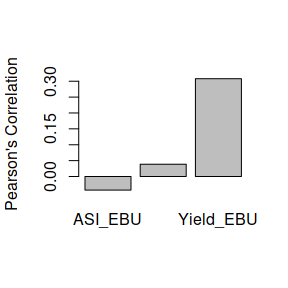# Fit the BMORS model for one environment of Maize dataset

#### 2019-02-11

This example shows how to evaluate prediction performance with multiple environments and traits in one single environment, using a BMORS_Env function. To do this, the MaizeToy dataset is used which is also available in the BMTME package, and it is loaded with the data() function as shown below:

library(BMTME)
data('MaizeToy')

With this command, you load the following 2 objects into the R environment: - genoMaizeToy: Genomic matrix of the dataset. - phenoMaizeToy: Phenotypic data from the dataset.

Remember that the first step that we need do, is order the dataset as follows:

phenoMaizeToy <- phenoMaizeToy[order(phenoMaizeToy$Env, phenoMaizeToy$Line),]

The model to be implemented must be defined and next the matrix design required are obtained with the following code:

LG <- cholesky(genoMaizeToy)
ZG <- model.matrix(~0 + as.factor(phenoMaizeToy$Line)) Z.G <- ZG %*% LG Once the matrix design has been generated, the linear predictor is constructed, for this purpose, a new object is created that we call ETA that consist of a series of nested lists as shown below, ETA <- list(Gen = list(X = Z.G, model = 'BRR')) Next, we obtain the dataset with the first column with the environments and the others are the phenotypic matrix, this can be done with the following code, dataset <- phenoMaizeToy[, 2:5] #Must Include in the first column the environments Finally, the model will be implemented, and for demonstration purposes only 2000 iterations are used to fit the model. In addition, we will show the resulting predictive performance using the summary() function. For this example, we only use the environment EBU to check the predictive capacities of the model and use the covModel = 'BRR' to specify the Bayes model to be used, by default is Bayes Ridge-Regression, so this parameter could be omitted. pm <- BMORS_Env(dataset, testingEnv = 'EBU', ETA = ETA, covModel = 'BRR', nIter = 2000, burnIn = 1000, thin = 2, progressBar = TRUE, digits = 3) summary(pm) #> Environment Trait Pearson MAAPE #> 1 EBU Yield 0.3079 0.1876 #> 2 EBU ASI -0.0428 0.3844 #> 3 EBU PH 0.0386 0.0696 To obtain a graph with the Pearson´s correlation or the model we use the barplot() function, as shown below, s barplot(pm)In this figure we can observe that the lowest Pearson´s correlation obtained was observed in the ASI_EBU trait-environment, while the highest Pearson´s correlation obtained in the predictive capacity is observed in the Yield_EBU trait-environment. If we need recover the predicted values, we could call the results from the object. head(pm$results, 5)
#>   Position Environment Trait Observed Predicted
#> 1        1         EBU Yield     6.88     5.474
#> 2        2         EBU Yield     6.85     5.800
#> 3        3         EBU Yield     6.37     6.006
#> 4        4         EBU Yield     4.98     6.573
#> 5        5         EBU Yield     7.07     5.844

Where the Position column, corresponds to the original position in the dataset.